# Special relativity using inertial frame moving at 1/2u

ChemicalCarl
Quick disclosure, I'm only a few weeks into modern physics, and still trying to get a firm grip on the mechanics involved here. My understanding is that it is possible to use a reference frame that is moving, and this leads to a bit of a paradox, at least under certain conditions.

Take two objects, A and B. A is stationary, and B is in motion with velocity v in the positive x direction. Initial conditions are tA=tB=xA=xB=0.

From the way special relativity has been described to me, it is allowable to take a reference frame that is stationary with object A, or a reference frame that is moving with velocity v along with object B.

If the reference frame is instead set to move at $\frac{1}{2}$v, this would cause the equation for time dilation to be t'=$\gamma$t for object B, with velocity $\frac{1}{2}$v. The same equation would apply for object A, but with velocity -$\frac{1}{2}$v.

Setting these two equations equal, you show that time passes equally for objects A and B. I posed this problem to my professor, who told me to read about the twin paradox in my book. I did so, and it failed to answer any questions in a mathematical way, or even definitively in a logical way. Further research hasn't clarified this for me (it's given me more questions!) The closest I've come to an answer to this question was "The short answer is yes, you are correct, and the long answer is general relativity."

I'm one of those learners who has issue with accepting canon and learning half-truths, but I think GR is still a bit above me at this point. Any elucidation would be greatly appreciated!

Carl

I don't see a twin scenario at all. In a frame with A stationary, B's clock is slow; in a frame with B stationary, A's clock is slow; in your frame where A and B move in equal and opposite directions (call this frame C), their clocks go at equal rates, both slower than C's clocks (but not by as much as A relative to B, or B relative to A).

This is the way relativity works: The speed of clock relative to an observer determines how the observer sees the clock's rate.

Before bringing in twin's what part of this is bothering you?

Last edited:
Mentor
Setting these two equations equal, you show that time passes equally for objects A and B.
There is no objective passage of time. For both observers, clocks at the other observers run slower. This is a perfectly valid statement, it is just not in agreement with human intuition.

You don't need general relativity here, as there is no gravity involved.

ChemicalCarl
The professor said general relativity was needed because acceleration would have to be involved to synchronize and later to compare the clocks. I guess I'm just not grasping how time passes faster for one and slower for the other in one frame, but by changing the reference frame the opposite is true. The twin paradox explanation in the book said that the person on earth has to be the stationary object, as the inertial frame would have to decelerate/accelerate to stay with the person traveling, in order to make the return trip.

I hate to pester with general questions about the twin paradox, as I know much has been written about it, but after a few days of racking my brain, it still doesn't truly 'click'.

Staff Emeritus
Gold Member
I don't know if this will help, But:

The thing about SR is that it forces us to deal with space and time in away that we are not used to. To explain how, I'm going to use an analogy.

Imagine that we plot time and space as two axis crossing each other at a right angle. In addition we will assume that the time axis is in the North-South direction and the space axis is in the East-West direction.
No matter what our orientation is to this plot, time is always North-South and Space is East-West. Pick any two points on the graph, and everyone agrees which one is to the North of the other.

This is how space and time was thought of before Relativity, space and time were objective.

What SR does, is replace this with space-time. With space-time, there is no objective direction that denotes time or space. It is like we have replaced North-South and East-West with Left-Right and Front-Back. As a result the "direction" of time differs for observers with different orientations to each other.

If you take the same two points as above, one observer can say that one point is to the left of the other, while another will say that it is the other way around, while a third says that neither is further to the left of the other.

This is like your problem, where A and B each measure each others clocks as running slow, while the third frame says that they run at the same speed (although slower than his own).

The professor said general relativity was needed because acceleration would have to be involved to synchronize and later to compare the clocks. I guess I'm just not grasping how time passes faster for one and slower for the other in one frame, but by changing the reference frame the opposite is true. The twin paradox explanation in the book said that the person on earth has to be the stationary object, as the inertial frame would have to decelerate/accelerate to stay with the person traveling, in order to make the return trip.

I hate to pester with general questions about the twin paradox, as I know much has been written about it, but after a few days of racking my brain, it still doesn't truly 'click'.

If you want good responses, you need to clearly (and not over verbosely) describe what you are asking about. Your initial post said nothing about turning around and getting back together. I take you at your word for what you're are asking.

However, even if acceleration and turning around were involved, there is absolutely no need for general relativity. This scenario was analyzed by numerous scientists using only special relativity within a couple of years of publication Einstein's 1905 paper (it was even covered briefly in Einstein's 1905 paper). Your professor is unfortunately promulgating an oft repeated inaccuracy.

If you want to wrestle with the twin paradox, read the following carefully, think about it, then come back with specific points of remaining confusion.

Last edited:
Mentor
I guess I'm just not grasping how time passes faster for one and slower for the other in one frame, but by changing the reference frame the opposite is true.

In the problem as you originally stated it, with no turnaround and and a constant relative velocity between the frames, you can resolve this apparent contradiction if you also consider the relativity of simultaneity.

When A says "B's clock is running slow", he is really saying "At the same time that my (A's) clock read one hour B's clock read .86 hours, therefore B's clock is slower than my (A's) clock".

However, because of the relativity of simultaneity, B does not agree that the the two events "A's clock reads one hour" and "B's clock read .86 hours" happened at the same time. as far as B is concerned, the correct statement is "At the same time that my (B's) clock read .86 hours, A's clock read .74 hours, therefore A's clock is slower than my (B's) clock". That is, to A the events "one hour on A's clock" and ".86 hours on B's clock" are simultaneous, but to B the events ".74 hours on A's clock" and "86 hours on B's clock" were simultaneous.

Thus, they both correctly claim that the other clock is running slow.

Gold Member
Quick disclosure, I'm only a few weeks into modern physics, and still trying to get a firm grip on the mechanics involved here. My understanding is that it is possible to use a reference frame that is moving, and this leads to a bit of a paradox, at least under certain conditions.

Take two objects, A and B. A is stationary, and B is in motion with velocity v in the positive x direction. Initial conditions are tA=tB=xA=xB=0.
The quickest, easiest and best way that I know of to understand Special Relativity is to learn how to do Lorentz Transformations and to plot your scenarios on spacetime diagrams representing Inertial Reference Frames (IRF's). And the LT's are easiest to do if you use units where the speed of light is equal to 1 so you don't have to carry around the factor of c. In this example, I use units of feet for distance and units of nanoseconds for time and I define the speed of light to be equal to 1 foot per nanosecond. I will call your objects "observers" because we will want to see what they can observe and how they make measurements.

We start with the spacetime diagram for an observer stationary in a frame. I just show a short period of time: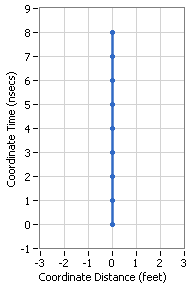Note that we commonly make time go up and the progress of time for the observer is shown by the dots along his worldline.

Now we want to see what it will look like if the observer were traveling at some speed v. I will use a speed of 0.6c which is the same as 6 feet in 10 nsecs. To do this, we transform the coordinates of the events (dots) of the scenario to a new speed of -0.6c: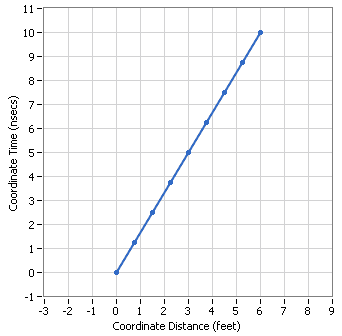Now you will note that time for the observer has been stretched out or dilated with respect to the coordinate time of this IRF.

Next we want to add in another observer who is stationary in this IRF: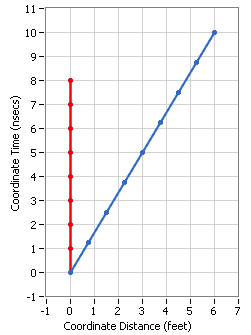Now we are ready to deal with your scenario. Observer A is depicted in red and observer B is depicted in blue.

From the way special relativity has been described to me, it is allowable to take a reference frame that is stationary with object A,
The way that these observers determine what is going on around them is to use radar signals that work like a laser rangefinder plus can be used for determining relative velocity. We assume also that they can observe the time on the other observer's clock as well as their own. We assume that both observers have set there own clocks to zero at the start of the scenario. You will have to count the dots to see what time is on their own clocks at each location.

Now I'm going to add in some radar signals, traveling at the speed of light (along 45-degree diagonals). Each observer sends out a radar signal at their own time of 1 nsec and observes that the reflection gets back to them at their own time of 4 nsecs (count the dots for the blue observer). They also observe that the other ones clock was displaying 2 nsecs when the reflection occurred: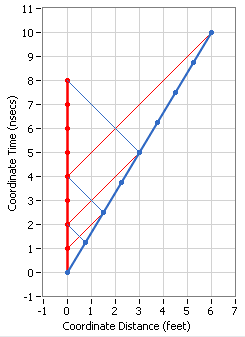So here's what they do with the information: They assume that the "time" of the radar measurement was the average of the "sent" time and the "received" time, in this case (1+4)/2 = 5/2 = 2.5 nsecs. And they assume that the relative distance to the other observer was the difference between those same two times divided by 2, in this case (4-1)/2 = 3/2 = 1.5 feet. And remember the time on the other observer's clock was 2 nsecs.

Meanwhile, they have sent out another radar signal at their own time of 2 nsecs which they each receive when their own time was 8 nsecs and 4 nsecs was observed on the other ones clock.

And as before, they determine the time of the second radar measurement to be (2+8)/2 = 10/2 = 5 nsecs and they determine the relative distance to the other observer to be (8-2)/2 = 6/2 = 3.0 feet.

Now they are ready to calculate the time dilation of the other ones clock. They do it by taking the difference in the determined times of the radar signals divided by the difference in the observed time on the others clock, in this case it would be (5-2.5)/(4-2) = 2.5/2 = 1.25.

They can also measure the relative speed of the other observer by taking the difference in the determined relative distance divided by the difference in the determined time difference. That would be (3-1.5)/(5-2.5) = 1.5/2.5 = 0.6.

All these observations/assumptions/calculations were done in observer A's rest frame and you can easily see that the distances, times, and velocity all comport exactly with the diagram for his rest frame. But observer B was also doing all the same observations/assumptions/calculations and he can do the same determinations (in a mirror image) but the above diagram does not agree with his determinations.

or a reference frame that is moving with velocity v along with object B.
Now we transform to the rest frame of observer B and we can see that all of his determinations agree with the diagram: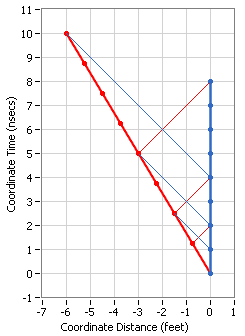If the reference frame is instead set to move at $\frac{1}{2}$v, this would cause the equation for time dilation to be t'=$\gamma$t for object B, with velocity $\frac{1}{2}$v. The same equation would apply for object A, but with velocity -$\frac{1}{2}$v.

Setting these two equations equal, you show that time passes equally for objects A and B. I posed this problem to my professor, who told me to read about the twin paradox in my book. I did so, and it failed to answer any questions in a mathematical way, or even definitively in a logical way. Further research hasn't clarified this for me (it's given me more questions!) The closest I've come to an answer to this question was "The short answer is yes, you are correct, and the long answer is general relativity."
You asked if we could pick a speed "half-way" between rest and observer B's speed in order to have both observers traveling in opposite directions at the same speed but this works at a speed somewhat higher than half-way, in this case 0.333c and here is a diagram depicting that: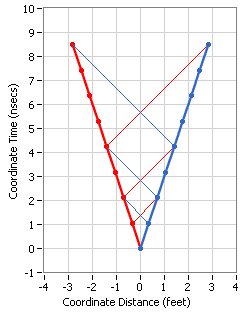I'm one of those learners who has issue with accepting canon and learning half-truths, but I think GR is still a bit above me at this point. Any elucidation would be greatly appreciated!

Carl
Hopefully this elucidation will help you see how in SR an observer can make measurements and after making the assumption that the speed of light is the same in both directions for him, he can make calculations that are independent of the frame in which the scenario is depicted but reflect the details for the frame in which he is at rest.

Any questions?

#### Attachments

Last edited:
•1 person
Gold Member
The professor said general relativity was needed because acceleration would have to be involved to synchronize and later to compare the clocks.
As others have pointed out, General Relativity is not needed as long as there is no gravity involved or if we can ignore the effects of gravity because they are much smaller than the effects from relative motions. In my previous post, there was no acceleration involved but now we want to consider the Twin Paradox and even though acceleration is involved, we can still analyze it entirely with Special Relativity.

I guess I'm just not grasping how time passes faster for one and slower for the other in one frame, but by changing the reference frame the opposite is true.
Did my previous post help? One of the important points that I was trying to make was that even though different frames assign the passage of time differently for each observer with respect to each frame, the observers see no difference whatsoever in the different frames. They can still make the same measurements, make the same assumption about the speed of light for themselves, and make the same calculations with regard to the other observer. Since that scenario was symmetrical, the answers they get are identical (except applied in the opposite direction).

The twin paradox explanation in the book said that the person on earth has to be the stationary object, as the inertial frame would have to decelerate/accelerate to stay with the person traveling, in order to make the return trip.
We can assume that the person on earth remains stationary and analyze the scenario using his inertial rest frame and have the other person travel away and back. But we can also transform to the inertial frame in which the traveler is inertial on his way out and then to another frame in which he is inertial on his way back and we can even construct a non-inertial frame in which the traveler is at rest for the entire trip. We do this using exactly the same radar method plus observing the other ones clock that I described in my previous post.

I'm going to start with the same parameters that I used in the previous post. The red worldline will represent the inertial twin that remains stationary in his rest frame while the blue worldline will represent the traveler who turns around after 8 nsecs of his time and returns in 8 more nsecs of his time. (If the very short trip disturbs you, just change the time units to years and the distance units to light-years.):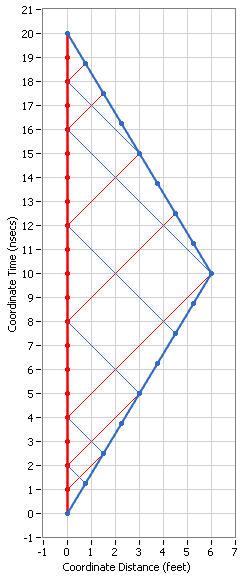Note how each observer continues to send out radar signals and receive their reflections but this time the new observations diverge.

Here is a summary of the measurements and calculations that the red observer makes of the blue observer's distance as a function of red's time, including the ones from the previous post plus his new ones:
Code:
Radar   Radar   Calculated   Calculated    Blue's
Sent    Rcvd      Time        Distance      Time
1       4        2.5           1.5          2
2       8        5             3            4
4      16       10             6            8
12      18       15             3           12

Note how his calculations exactly match what his rest frame depicts including the simultaneity of Red's Calculated Time and Blue's Time. (You have to count Blue's dots to see this.)

But now we want to do the same thing for the blue observer calculating the position of the red observer:
Code:
Radar   Radar   Calculated   Calculated    Red's
Sent    Rcvd      Time        Distance     Time
1       4        2.5           1.5         2
2       8        5             3           4
4      10        7             3           8
6      12        9             3          12
8      14       11             3          16
12      15       13.5           1.5        18
Now it's obvious that Blue's calculations of Red's position does not match the above frame but let's see what happens if we transform to Blue's rest frame during the outbound portion of his trip: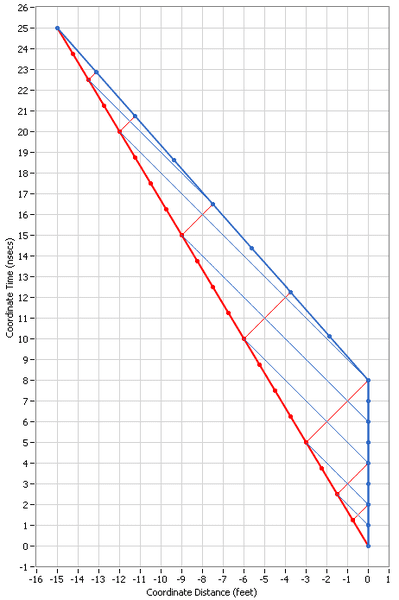This is definitely a step in the right direction as it agrees with the first two calculations of Red's positions but not after that.

Let's try the frame in which Blue is at rest during the inbound portion of his trip: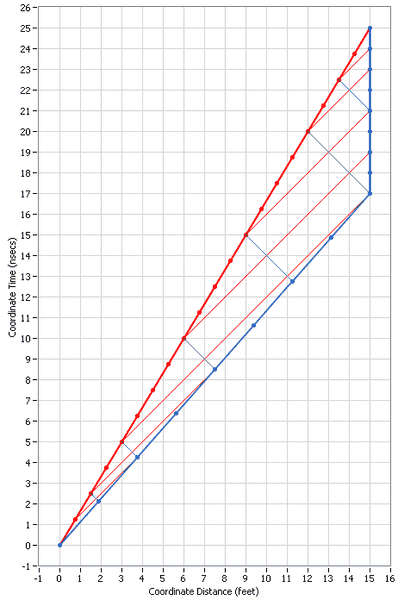Now this works for the last two calculations of Red's positions but not before.

It turns out that since Blue is not inertial we cannot transform to a frame in which Blue is at rest but we can construct one based on the information in the table. Here's what it looks like: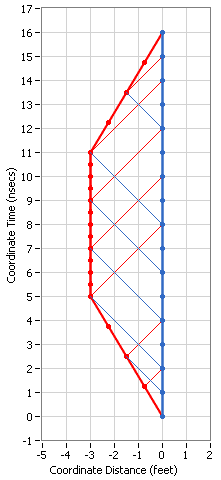You should be able to see that the bottom triangular portion of this frame matches exactly the bottom of the scenario in the second frame above and the top triangular portion of this frame matches exactly the top of the scenario in the third frame above. So all we have to do is fill in the remaining triangular portion in the middle as shown in the above diagram.

Now you should be able to see that this non-inertial rest frame for the blue observer matches all of his measurements, observations and calculations that he made earlier and compiled in the second table above. And, as always, all of the diagrams show the same observations for both observers.

Since this thread is about using an inertial frame in which both observers are traveling away from each other at the same speed, I'm going to show you two more diagrams in which this is true for a portion of the scenario. Here is the one for the first portion of the scenario: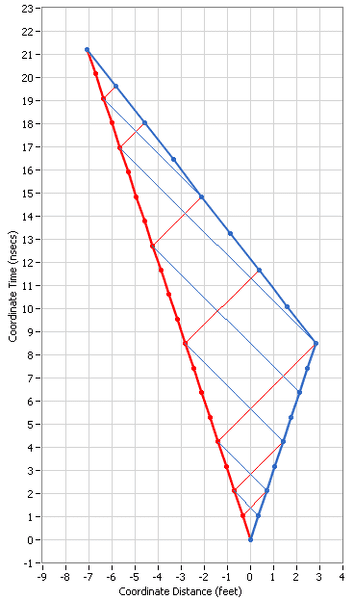And here is the one for the last portion for the scenario: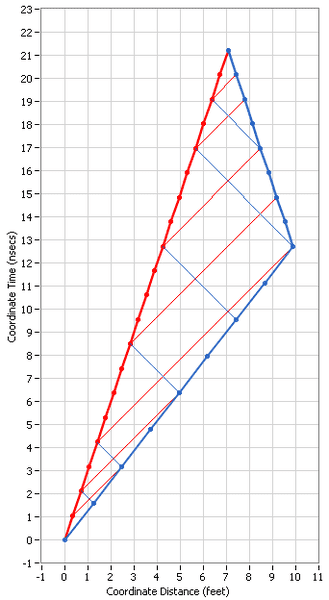But note that it is not possible to combine the two portions of these two diagrams where the observers are moving at the same speed, at least I don't know how it could be done.

Any questions?

I hate to pester with general questions about the twin paradox, as I know much has been written about it, but after a few days of racking my brain, it still doesn't truly 'click'.

#### Attachments

dauto
I like those diagrams. What software did nyou use to create them?

ChemicalCarl
Thank you all for the help! I think I'm grasping things much better now. I waited a bit to respond as I wanted to digest all this info properly.... it seems this is one of those fields that just takes awhile to sink in. The graphs were extremely helpful, by the way, ghwellsjr! Thanks!

Now, onwards to quantum, this modern physics class sure does move fast! It's a pity, SR has been one of my favorite subjects to learn thus far. I have a feeling I'll continue to creep around on this board. ;)

Thanks again,
Carl